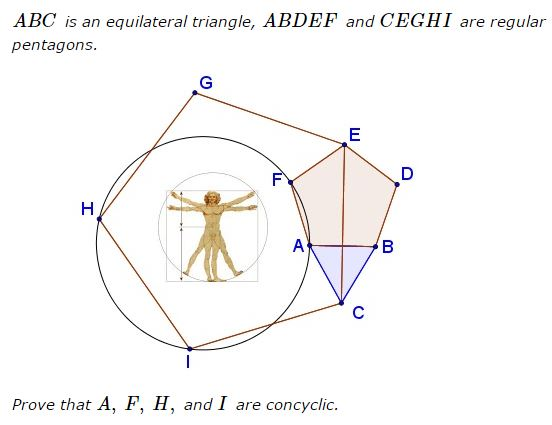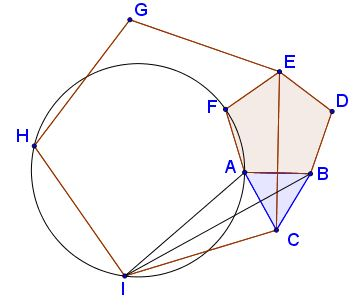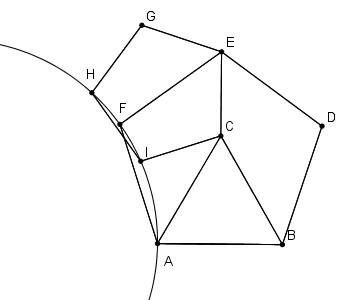# K. Knop's Problem with Two Regular Pentagons And an Equilateral Triangle

### Problem### Solution 1

Solution proceeds in several steps. First prove $BI\perp AC\,$ which implies $IA=IC:\,$such that

$\angle CAI=\angle ACI=108^{\circ}-30^{\circ}=78^{\circ}.$

Let $J\,$ be the center of the circle $(AHI):$Since $\angle CIA=180^{\circ}-2\cdot 78^{\circ}=24^{\circ},\,$ $\angle AIH=108^{\circ}-24^{\circ}=84^{\circ}.\,$ $IH=IC=IA,\,$ implies $\Delta AIJ=\Delta IJH,\,$ from which $\angle AIJ=\angle JIH=\frac{1}{2}\angle AIH=42^{\circ}.\,$ Further, since $\Delta AIJ\,$ is isosceles, $\angle IAJ=\angle AIJ=42^{\circ}.\,$ By additional angle chasing, $\angle BAI=78^{\circ}+60^{\circ}=138^{\circ},\,$ so that $\angle IAJ+\angle BAJ=180^{\circ}\,$ and $BAJ\,$ is a straight line.

On the other hand, $EF\,$ being the bisector of $\angle CEG,\,$ $EF\perp HI\,$ and passes through the midpoint $K\,$ of the latter.Since the $J\,$ lies on the perpendicular bisector of $HI,\,$ $J\in EK\,$ so that $J\,$ is the intersection of the $AB\,$ and $EF,\,$ extended, which obviously implies that $FJ=AJ,\,$ placing $F\,$ squarely on the circle $(FHI).$

Now, to return to the beginning. The only way I can prove that $BI\perp AC\,$ is by trigonometry.Taking $C\,$ for the origin, with horizontal $x-\text{axis}\,$ and vertical $y-\text{axis}\,$ and assuming $AB=2,\,$ we get $\left(\displaystyle \varphi=\frac{\sqrt{5}+1}{2}\right):$

\displaystyle \begin{align} &B=(1,\sqrt{3}),\\ &IC=CE=\sqrt{3}+2\cdot\varphi\cdot\cos 18^{\circ}=\sqrt{3}+(\sqrt{5}+1)\frac{\sqrt{2(5+\sqrt{5})}}{4},\\ &\angle CIL=18^{\circ}\,\Rightarrow\,I=IC\cdot(-\cos 18^{\circ},-\sin 18^{\circ}),\\ &\tan\angle BIL=\frac{\sqrt{3}+IC\cdot\sin 18^{\circ}}{1+IC\cdot\cos 18^{\circ}}. \end{align}

Due to the lack of patience and being sure there ought to be a synthetic shortcut, I ran this ratio by wolframalpha which produced $\displaystyle \frac{1}{\sqrt{3}}\,$ as one of alternative results. Thus, $\tan\angle BIL=\displaystyle \frac{1}{\sqrt{3}},\,$ giving $\angle BIL=30^{\circ},$ as required.

### P.S.

Here's a direct proof that $BI\perp AC.\,$ We write $M\,$ for the midpoint of $AC\,$ and $\tan(60^{\circ})=\sqrt{3},\,$ $\tan(72^{\circ})=t\,$ so that $1+t^2 = 4\varphi^2,\,$ and, thus, $t^2-3 = 4 \varphi^2 - 4 = 4\varphi.\,$ Suffice it to show that $\Delta ICM\,$ is right-angled at $M,\,$ that is, that $\csc(12^{\circ}) = t+\sqrt{3}.$

Proof:

\displaystyle \begin{align} (t+\sqrt{3})^2 &= \left(\frac{4\varphi}{t-\sqrt{3}}\right)^2\\ &= 4\frac{1+t^2}{(t-\sqrt{3})^2}\\ &= 1 + \left(\frac{1+t \sqrt{3}}{t-\sqrt{3}}\right)^2\\ &= 1+ \cot^2(72^{\circ}-60^{\circ})\\ &= \csc^2(12^{\circ}). \end{align}

### Solution 2

Second proof of the problem (the other way round). We start from a regular pentagon $HICEG.\,$ Consider the circular arc formed (on the same side of line $HI\,$ as $E)\,$ by the points from where $HI\,$ subtends an angle of $48^{\circ}.\,$ Let $J\,$ be the center of this circle, $F\,$ the intersection of the circular arc with the perpendicular bisector of $HI,\,$ $A\,$ the point of the arc on the perpendicular to $CE\,$ through $J,\,$ and $B\,$ and $D\,$ the reflections of $A\,$ and $F\,$ across $CE.$Simple angle chasing shows that $IA=IC,\,$ $\angle CEF = 54^{\circ},\,$ $\angle EFA = \angle FAB = 108^{\circ},\,$ and $\angle BAC = 60^{\circ}.\,$ We are done if we show that $EF=FA:\,$ then we have a regular pentagon $ABDEF\,$ and an equilateral triangle $ABC.$

Proof of $EF=FA,\,$ that is, $\displaystyle \cot(18^{\circ})-\cot(24^{\circ}) = 2\frac{\sin(18^{\circ})}{\sin(48^{\circ})}\,$. We use the fact that $s=\sin(18^{\circ}),\,$ being equal to $\displaystyle \frac{\varphi - 1}{2},\,$ has the property $\displaystyle 2 s^2 = \frac{1}{2} - s.\,$ Thus

\displaystyle\begin{align} \cot(18^{\circ})-\cot(24^{\circ}) &= \frac{\sin(6^{\circ})}{s\sin(24^{\circ})} = \frac{2\sin(6^{\circ})\cos(24^{\circ})}{s\sin(48^{\circ})}\\ &=\frac{\sin(30^{\circ})-s}{s\sin(48^{\circ})} = \frac{2 s^2}{s\sin(48^{\circ})} = \frac{2 s}{\sin(48^{\circ})}. \end{align}

and we are done.

### Acknowledgment

The problem has been posted at the Οι Ρομαντικοι της Γεωμετριας (Romantics of Geometry) facebook group by Konstantin Knop.

Konstantin has requested not to leave out a similar problem:I do leave it as an exercise to the reader. It's the same circle, though.Grégoire Nicollier has come up with a direct proof (P.S.) of $BI\perp AC.$ Konstantin has also added an extra point to the circle on observed that, be symmetry, points C, B, E, O, N are also concyclic.Grégoire Nicollier has later added another proof (Solution 2), a variant of which has been placed on a separate page.